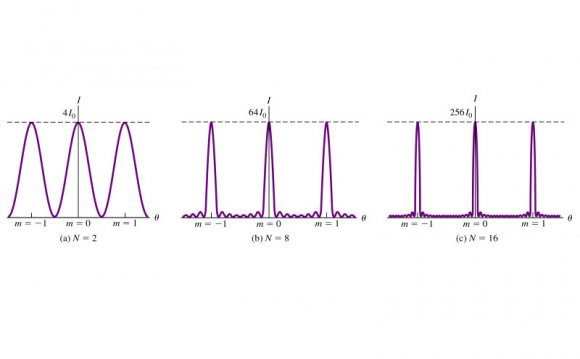# What is diffraction grating in Physics?

November 3, 2020Reference: Carroll, Bradley W. & Ostlie, Dale A. (2007), An Introduction to Modern Astrophysics, 2nd Edition; Pearson Education – Chapter 5, Problem 5.2.

Jenkins, Francis A. & White, Harvey E. (1957), Fundamentals of Optics, 3rd Edition; McGraw-Hill – Chapter 17.

One of the main sources of information about celestial objects is their spectrum. In the case of light from a star, the spectrum can tell us a lot about the composition of the star, and the Doppler shift can tell us the star’s radial velocity.

Getting a detailed spectrum of a star is somewhat more involved than just passing its light through a prism, as Newton famously did to show that white light is composed of the colours of the rainbow. In practice, a diffraction grating is used to split starlight into its constituent spectral lines. A complete treatment of the physics of diffraction gratings is beyond the scope of this post, so if you’re interested I’ll refer you to any good textbook on optics. [My own reference, the book by Jenkins and White mentioned at the top, is quite dated now, but I doubt that the physics has changed in the intervening years.] I’ll give a summary of the key concepts here.

A diffraction grating is basically a screen with a large number of closely-spaced parallel slits cut in it (actual gratings are usually made of glass with very fine lines ruled on them, but the principle is the same). Consider a grating with only 2 slits separated by a distance . Monochromatic light of wavelength is shone onto the grating and diffracts through each of the slits. [Diffraction is the process by which a light wave spreads out after passing through a narrow gap. The same effect can be seen with water waves as they hit a narrow gap in a barrier; waves spread out in a semi-circular pattern beyond the gap.]

Now look at the light rays that leave the slits at an angle to the normal to the plane containing the slits. By drawing a diagram (see Fig 3.3 in Carroll & Ostlie, although in practice the two light rays leaving the slits are parallel and are focussed onto the detecting screen by a convex lens or concave mirror) we can see that the path difference between the two rays is . If this path difference is an integral multiple of the wavelength, the two rays will reinforce each other and we’ll see a bright fringe at that angle. On the other hand, if the path difference is an integral multiple of wavelengths plus half a wavelength, the two rays will destructively interfere, cancelling each other out, and we’ll see a dark fringe. That is, the condition for bright fringes is

for a non-negative integer.

Now suppose we have a diffraction grating with slits. The same condition applies for light fringes, but because we’re now adding up rays instead of just 2, the intensity of the bright fringes is larger, and is in fact proportional to . The actual formula for the intensity is

where is a constant, derived from the intensity passing through a single slit, and

The bright fringes occur when . This can be seen by finding the limit:

This limit can be found using l’Hôpital’s rule from calculus, which states that for two functions and where :

Therefore

Because of the in the upper sine, however, the numerator has many more zeroes than the denominator, meaning that whenever but is not a multiple of . Between any two bright fringes there will therefore be dark fringes and secondary ‘brightish’ fringes between these dark fringes. In practice, these secondary fringes are much fainter than the primary bright fringes and for large they are effectively invisible.

It turns out that the number of slits also determines the angular width of each primary maximum, according to

That is, the more slits, the sharper the spectral line for a given wavelength. Looked at another way, the smallest wavelength difference that can be resolved is

Not only does increasing the number slits increase the resolving power of the grating, but looking a higher order (larger ) lines allows greater resolution.

Example The sodium D lines are commonly found in stellar spectra and have wavelengths of and . If light containing these two lines shines on a grating with 300 lines per millimetre, then

From with (second-order spectra) we have

The angle between the two lines is therefore

From we can work out how many lines need to be illuminated in order for these 2 lines to be resolved in second-order. For the wavelength in the formula, we’ll use the average of the two wavelengths of the sodium D lines.

Thus if only 2 mm of the grating were illuminated, we could resolve the lines, at least at second-order.

Source: www.physicspages.com
##### RELATED VIDEOPhysics: Interference and diffraction (13)Diffraction GratingDiffraction Grating Interference